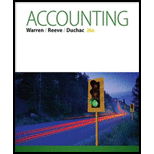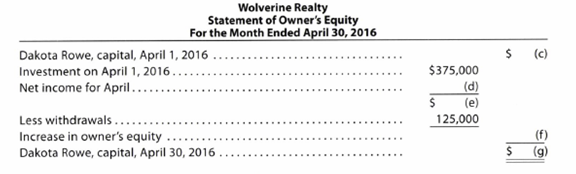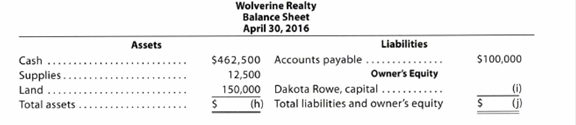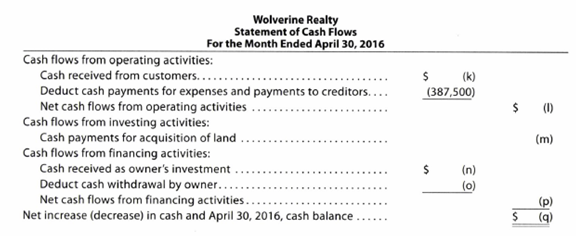Chapter 1, Problem 1.6APR### Accounting (Text Only)

26th Edition
Carl Warren + 2 others
ISBN: 9781285743615

#### Solutions

Chapter
Section### Accounting (Text Only)

26th Edition
Carl Warren + 2 others
ISBN: 9781285743615
Textbook Problem
1 views

# Missing amounts from financial statementsThe financial statements at the end of Wolverine Realty’s first month of operations are as follows:InstructionsBy analyzing the interrelationships among the four financial statements, determine the proper amounts for (a) through (q).

To determine

Financial statements:

Financial statements refer to those statements, which are prepared by the Company according to particular formats in accounting to show its financial position.

Financial statements include the following statements:

Income statement:

Income statement is a financial statement that shows the net income or net loss by deducting the expenses from the revenues and vice versa.

Statement of owner's’ equity:

This statement reports the changes in the owners’ equity for a particular period of time

Statement of cash flows:

This statement reports all the cash transactions which are responsible for inflow and outflow of cash, and result of these transactions is reported as ending balance of cash at the end of reported period.

To Determine:  The missing amount in the given financial statement of Company WR.

Explanation

Calculate the missing amounts in the given financial statement of Company WR for month ended April 30, 2016.

 Company WR Income Statement For the month ended April 30, 2016 Particulars Amount ($) Amount ($) Revenues Fees earned (a)  $750,000 Expenses Wages expense$300,000 Rent expense $100,000 Supplies expense (b)$30,000 Utilities expense $20,000 Miscellaneous expense$25,000 Total expenses $475,000 Net income$275,000

Table (1)

 Company WR Statement of Owner's Equity For the month ended April 30, 2016 Particulars Amount ($) Amount ($) DR Capital, April 1, 2016 (c)   $0 Investment on April 1,2016$375,000 Net income for the month (d)   $275,000 Changes before withdrawals (e)$650,000 Less: Withdrawals $125,000 Increase in Owner's equity (f)$525,000 DR Capital, April 30, 2016 (g)   $525,000 Table (2)  Company WR Balance Sheet April 30, 2016 Particulars Amount ($) Amount ($) Assets Current Assets Cash$462,500 Supplies $12,500 Land$150,000 Total current assets (h)   $625,000 Liabilities and Stockholders’ Equity Liabilities Accounts payable$100,000 Owner's equity DR Capital (i)   $525,000 Total liabilities and stockholders’ equity (j)$625,000

Table (3)

 Company WR Statement of Cash Flows For the month ended April 30, 2016 Particulars Amount ($) Amount ($) Cash flows from operating activities: Cash receipts from customers (k)    $750,000 Cash payments for expenses and creditors$387,500 Net cash flow used for operating activities (l)      $362,500 Cash flows from investing activities: Cash payment for acquisition of land (m) ($150,000) Cash flows from financing activities: Cash receipt of owner’s investment (n)  $375,000 Cash Withdrawals (o)$125,000 Net cash flow from financing activities (p)  $250,000 Net Increase in cash, April 30,2016 cash balance (q)$462,500

Table (4)

Working Notes:

1. a) Fees Earned: Fees earned during the month of April is $750,000. Calculate the fees earned. Net Income = Revenue ExpenseRevenue = Net Income + Expense=$475,000 + $275,000=$750,000 (a)

2. b) Supplies expense: Supplies expense during the month of April is $30,000. Calculate the supplies expense. Expenses = [(WageExpense)+(RentExpense)+(SuppliesExpense)+(UtilitesExpense)+(MiscellaneousExpense)]$475,000=$300,000+$100,000+(SuppliesExpense)+$20,000+$25,000$475,000=$445,000+(Supplies Expense)Supplies Expense=$475,000$445,000=\$30,000 (b)

1. c) DR Capital, April 1, 2016:  As this is the first month of operation for Company WR, there will be having no opening balance for the Capital account

### Still sussing out bartleby?

Check out a sample textbook solution.

See a sample solution

#### The Solution to Your Study Problems

Bartleby provides explanations to thousands of textbook problems written by our experts, many with advanced degrees!

Get Started